# Binary Genetic Algorithm

In this article we will look at what a genetic algorithm is and how a Binary Genetic Algorithm works. We will walk through an example to understand the details.

Before we dive deep into how Binary Genetic Algorithm (BGA) works, we will first look into what it is used for. As the name suggests, Genetic Algorithms use the concept of genes from biology and are used to help optimise a problem that is formulated as an objective function. They can be used in optimising complex engineering problems in thermodynamics to optimising error rate in Neural Networks.

A function f(x) will give the minimum or maximum value for a certain optimised value of x, where x can be in a given range (e.g. -10<x<10). We can have functions that solve for multiple variables as well,

e.g. f(x,y,z) = 2x+3y+10z-3.6, where x,y,z can have values in a given range.

So, before selecting an optimisation algorithm, formulating the problem statement as a function is a key step.

Genetic Algorithms are a subclass of Evolutionary Computing and are population based optimisation methods. It is inspired by Darwin’s theory of evolution, which in a nutshell means “survival of the fittest”. As in real world, an offspring will have several characteristics of its parents and not every offspring may survive, BGA also discards offsprings that do not generate a good output. We shall look into this in more detail.

Let’s first look into the key terminologies used in BGA:

The decision variables are represented as Chromosomes = [P1, P2,…., Pn]

Each gene (Pi) in the chromosome is coded by m bits (binary representation). A single decision variable can be referred to as a gene.

BGA uses a group of chromosomes called Population (Npop) and the cost function can be represented as f(chromosome). The population goes through a series of updates or generations to evolve.

Consider an example where we have 3 decision variables attributing to a problem, and the cost function is f(x,y,z) = 2x+3y+10z-3.6, where each variable is coded by 10 bits:

Chromosome = 

here, the first 10 bits represent x, the second 10 bits represent y and the last 10 bits represent z.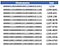Example: Population and cost of each Chromosome

The purpose behind generating a population is to collect a group of potential solutions, which will evolve to improve their quality in each generation.

A basic flow of the BGA process is as follows: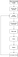Flow of a BGA process

Step 1: Define the cost function f(x), variables that are part of cost functions, and other GA parameters like number of bits per gene (m), population size (Npop), mutation rate (µ), selection process, mating process and convergence condition.

Step 2: Generate an initial population based on the range of decision variables and decided population size. In the example above, decision variables are x, y and z and let us assume population size as 12. For any given continuous values of the variables, they are first converted to binary to form the chromosomes and population. e.g.:

if the range for X = [25,36], Y = [39,50], Z = [91,102]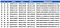Example: Population generation

Step 3: Decoding chromosomes in the population to represent the decision variables in the solution space. For e.g., 0000000000 is binary form for 0, but it does not fall under that solution space for X = [25,36], hence we use the below formula to decode the genes which further will be used in calculating the cost of a chromosome:

0000011010, X = (25) + decimal(0000011010) (36–25)/(1024–1) = 25.27

and 0000000000 will correspond to 25 in the solution space.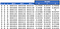Example: Decoding decision variables

Step 4: Once the decision variables have been decoded, calculate the cost of each chromosome and rank them in order, here minimising cost is the objective

Step 5: This step is referred to as Natural Selection, where a pair of chromosomes are selected to mate with each other to generate further offsprings in each generation. BGA first selects a set of fittest chromosomes (Nkeep) from which the parents will be selected and all other chromosomes are discarded and be replaced by generated offsprings. There are 2 ways in BGA for selecting Nkeep:

1. Xrate. Selection rate is a pre-defined fraction of the total population.
2. Thresholding. A threshold is set for the cost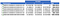Nkeep = Xrate * Npop | Xrate = 50% and Npop = 12, Nkeep = 6

Once Nkeep is obtained, parents (pair of chromosomes) are selected for mating. There are 4 approaches in BGA to select parents:

1. Pairing in sequential order: Select parents from top to bottom
2. Random Pairing: Generate random pairs for mating
3. Weighted Random Pairing: It includes Rank weighting and Cost weighting. The pairs are selected based on their probabilities.
4. Tournament Selection: Each pair of chromosome go through a series of tournament to find the best pair. In a tournament, a chromosome with the lowest cost wins.

In this article, we shall consider Rank weighting to select parents. We calculate the probability of each chromosome.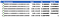Probability of each Chromosome

The probability distribution can be represented as below number lineProbability Distribution (Image not to scale)

Generate a set of random number for each pair to select parents. For e.g. the first 2 random numbers [0,1] are 0.321 and 0.765, we select P2 and P5 as parents. Similarly, we select all other parents, in total 3 pairs in this example.

Step 6: There are 3 key approaches to perform mating:

1. Single point Crossover
2. Double point Crossover
3. Uniform Crossover

In a single point crossover, a crossover point is randomly selected between the first and last bits of the chromosomes and the bits are swapped. For e.g. when P2 and P5 are selected for mating with single point cross over at 15th bit: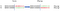Mating generates 2 offsprings using single point crossover

In Double point crossover, the bits exchanged between the parents are between 2 crossover points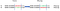Double point cross over

A uniform crossover consider random number generation and a threshold to select at each bit whether to exchange bit or not. It proves to be computationally expensive.

Referring to the example, once the 3 sets of offsprings are generated, using 3 sets of parents, the offsprings replace the discarded chromosomes in the original population.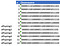Offsprings in population

Step 7: Mutation occurs in the population after the offsprings have been generated. It is a random process to mutate the bits in the population. It allows BGA to introduce new information in the population. If the new population is not effective in terms of cost, it will get discarded in the next generation or iteration. Mutation rate decided how many bits in the population will be mutated. The general formula to calculate is:

#mutation = µ*(Npop -1)*Nbits

Where, µ is the mutation rate and Nbits is the total number of bits in the chromosome. (Npop -1) refers to elitism where mutation is not performed on the first chromosome in the population. The value can be any number between 0 and Npop-1.

For e.g. if we select µ = 0.1, bits to be mutated in our population will be 33. In mutation we simply flip the bit value from 0 to 1 or vice versa for the selected bits.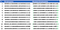Mutation on 33 bits in the Population

Step 8: Once the mutated population is generated, BGA checks for any given convergence conditions. If the conditions are met, process stops and we have the values for decision variables that potentially minimises the objective function. If the conditions are not met, BGA iterates through multiple generations and repeats the process of Decoding, Cost Calculation, Mating, and Mutation.

Below figure shows an example of minimisation of a cost function with every generation, where we can see that the best cost calculated drops to 0 after 10th iteration.

Conclusion:

BGA can be used to optimise with continuous as well as discrete variables. It is not necessary to have a differentiable cost function such as is needed in gradient descent algorithm. BGA can deal with a large number of decision variables and can optimise with complex functions. Key benefit of using BGA is, it is less likely to get trapped in local minimum and tends to search for global minimum. However, there is a high chance of information loss when dealing with continuous variables in BGA. For continuous variables it is preferable to use Continuous Genetic Algorithm or CGA. CGA uses the same concept as BGA except the decoding/ encoding step.

References:

 Title Image

 A genetic algorithm for function optimization: a Matlab implementation

Exploring how AI can benefit core engineering fields

## More from Prateek Mishra

Exploring how AI can benefit core engineering fields